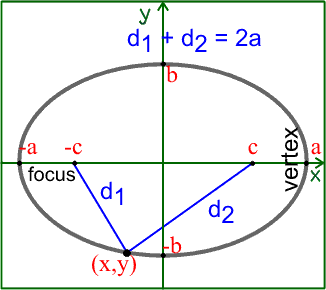## Labels

### Conic Section Vocabulary

curve
the trace of a point whose direction of motion changes. There is no better example of this than the work done by the ancient Greeks on the curves known as the conics: the ellipse, the parabola, and the hyperbola.

(geometry) a curve generated by the intersection of a plane and a circular cone

ellipse in which the two axes are of equal length

His work "Conics" was the first to show how all three curves, along with the circle, could be obtained by slicing the same right circular cone at continuously varying angles.

hyperbola
an open curve formed by a plane that cuts the base of a right circular cone

parabola
a curve formed by an object thrown in the air and falling

ellipse
a closed plane curve with an oval shape

center: the point (h, k) at the center of a circle, an ellipse, or an hyperbola.

vertex (VUR-teks): in the case of a parabola, the point (h, k) at the "end" of a parabola; in the case of an ellipse, an end of the major axis; in the case of an hyperbola, the turning point of a branch of an hyperbola; the plural form is "vertices" (VUR-tuh-seez).

focus (FOH-kuss): a point from which distances are measured in forming a conic; a point at which these distance-lines converge, or "focus"; the plural form is "foci" (FOH-siy).

directrix (dih-RECK-triks): a line from which distances are measured in forming a conic; the plural form is "directrices" (dih-RECK-trih-seez).

axis (AK-siss): a line perpendicular to the directrix passing through the vertex of a parabola; also called the "axis of symmetry"; the plural form is "axes" (ACK-seez).

major axis: a line segment perpendicular to the directrix of an ellipse and passing through the foci; the line segment terminates on the ellipse at either end; also called the "principal axis of symmetry"; the half of the major axis between the center and the vertex is the semi-major axis.

minor axis: a line segment perpendicular to and bisecting the major axis of an ellipse; the segment terminates on the ellipse at either end; the half of the minor axis between the center and the ellipse is the semi-minor axis.

 Circle EllipseParabola Hyperbola Variables: r = circle radius a = major radius (= 1/2 length major axis) b = minor radius (= 1/2 length minor axis) c = distance center to focus p = distance from vertex to focus (or directrix) a = 1/2 length major axis b = 1/2 length minor axis c = distance center to focus Eccentricity: 0 c/a c/a Relation to Focus: p = 0 a2 - b2 = c2 p a2 + b2 = c2 Definition: is the locus of all points which meet the condition... distance to the origin is constant sum of distances to each focus is constant distance to focus = distance to directrix difference between distances to each foci is constan